# Weyl method

(diff) ← Older revision | Latest revision (diff) | Newer revision → (diff)

in number theory

A method for obtaining non-trivial estimates of trigonometric sums (cf. Trigonometric sum) of the form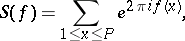(1)

whereandare arbitrary real numbers. Developed by H. Weyl  to establish criteria for uniform distribution (cf. Weyl criterion).

The method may be described as follows. The sum (1) is raised to the powerby successive squaring operations in order to reduce the degree of the polynomial. Thus, in the first stage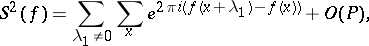where the summations are performed over intervals of lengths; now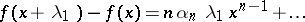is a polynomial of degree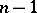in(the symbols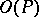,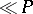denote magnitudes of order). At the-st step one obtains inner sum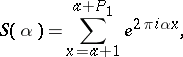(2)

where,,. Sums of the form (2) are estimated using the inequalityand the resulting estimate is:(3)The inequality (3) yields different estimates for the sum (1) in caseis small in comparison to. These estimates depend on the accuracy with which the coefficient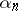of the polynomialcan be approximated by rational fractions.

Example. LetThen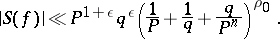In particular, ifthen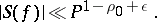Weyl's method gives solutions, to a first approximation, of several important problems in number theory. The estimate (3) and its corollaries were used to study the distribution modulo one of the polynomial. G.H. Hardy and J.E. Littlewood (1919) gave a solution of the Waring problem which was based on estimating the sums (1) by Weyl's method. They could thus estimate the values offor which the equationwhere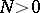is an integer and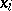are integers, is solvable, and even gave an asymptotic formula for the number of solutions. A generalization of the estimate (3) to the case of functionswhich are not polynomials but are in a certain sense close to polynomials, resulted in the improvement of certain theorems in the theory of the distribution of prime numbers (an estimate of the difference between two successive prime numbers and an estimate of the residual term in the asymptotic formula for the numberof prime numbers not exceeding).

The insufficient strength of the estimates obtained by Weyl's method is due to the high power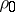to which the sumis raised. J. van der Corput proposed a somewhat improved method for estimating the sums (1). The Vinogradov method yields a very accurate upper bound for the integral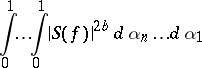already for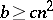(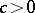a constant,). This estimate (cf. Vinogradov theorem about the average) may be used to deduce essentially new estimates of Weyl sums (1) (with reduction factor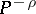,;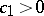a constant), which cannot be attained by Weyl's method.

How to Cite This Entry:
Weyl method. Encyclopedia of Mathematics. URL: http://encyclopediaofmath.org/index.php?title=Weyl_method&oldid=49204
This article was adapted from an original article by B.M. Bredikhin (originator), which appeared in Encyclopedia of Mathematics - ISBN 1402006098. See original article